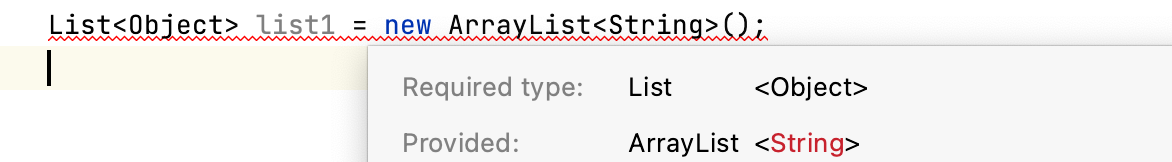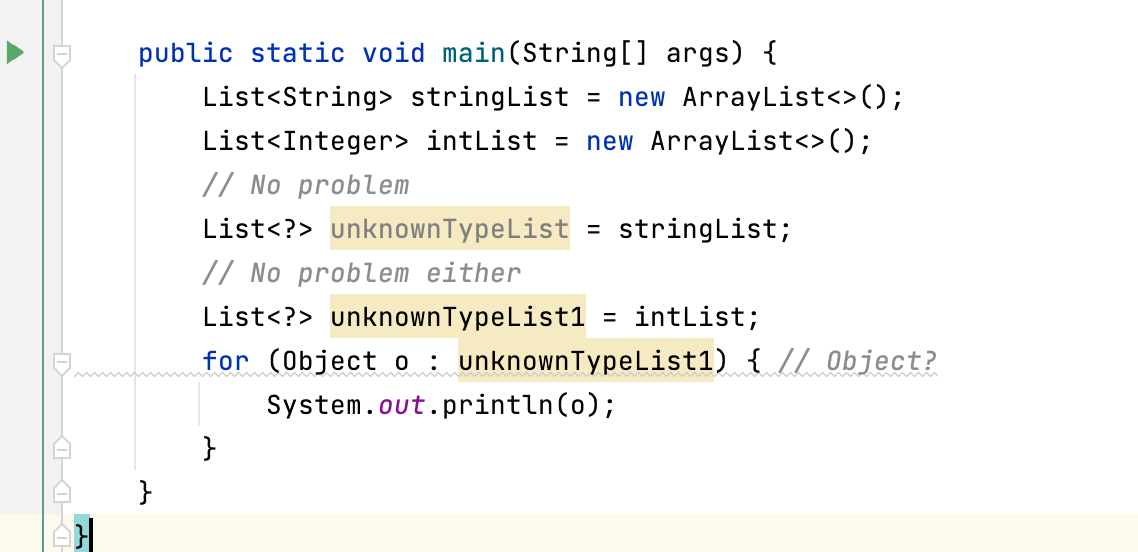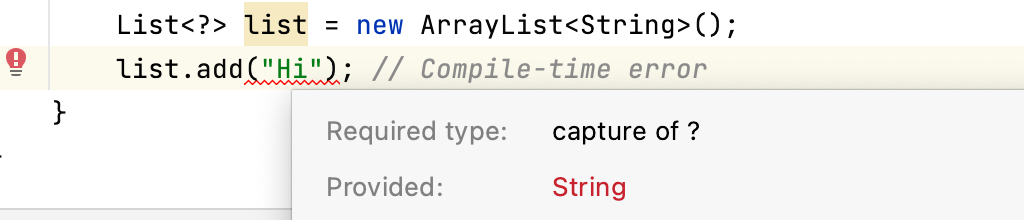# 范型

java5中添加了泛型作为类型检查的机制，提前告知类型，避免强转

• 泛型是编译器处理的，在运行时，Java 不知道泛型。编译器验证您使用的类型是否正确，然后使用 java.lang.Object类型
在运行时，List < string > 和 List < integer > 是相同的，因为类型信息已经被编译器删除了(它们只被看作 List)。且只能处理包装类型，不能处理基本类型

# 菱形<>语法

Java 7以来，不再在赋值的两边指定泛型类型

# 范型类

• List接口的源码
``````public interface List<E> extends Collection<E> {
Iterator<E> iterator();
}
``````

E只是一个标识符，就像一个命名的变量。它可以是你想要的任何东西。但是，约定是使用单个大写字母。

• 一些常见的字母:
E 元素
K 键
V 值
T 数据类型

## 范型好处举例

``````class Holder {
private String s;
public Holder(String s) {
this.s = s;
}
public String getObject() {
return s;
}
public void printObject() {
System.out.println(s);
}
}

class IntegerHolder {
private Integer s;
public IntegerHolder(Integer s) {
this.s = s;
}
public Integer getObject() {
return s;
}
public void printObject() {
System.out.println(s);
}
}
``````

``````class Holder<T> {
private T t;
public Holder(T t) {
this.t = t;
}
public T getObject() {
return t;
}
public void printObject() {
System.out.println(t);
}
}
``````
``````Holder<String> h1 = new Holder<>("Hi");
Holder<Integer> h2 = new Holder<>(1);
String s = h1.getObject();
``````

# 范型方法

``````class Utils {
public static <T> void print(T t) {
System.out.println(t);
}

<T> void genericMethod1(List<T> list) {
}

<T, U> T genericMethod2(U u) {
T t = null;
return t;
}
}
``````

# 通配符

• 范型的局限性
在使用泛型时，不能将派生类型分配给基类型; 两种类型应该是相同的(显式地或使用菱形运算符)。换个角度List < Object > 类型的列表可以保存 Object 及其子类的实例。换句话说，列表可以包含任何对象类型，而不仅仅是字符串。例如，您可以有一个字符串和整数的列表，这明显违反了类型安全。

## 无界通配符<?>• 因为编译器不能推断元素的类型，所以不能保证类型安全(只能插入 null，因为它没有类型)但是当使用在方法参数中时，这些方法的代码只使用泛型类的方法或者来自 Object 的方法，而不是特定类型的方法
``````// You can pass any type of List here
int getSize(List<?> list) {
return list.size();
}
``````

## 有界通配符? extends T ? super T

``````class Printer<T> {
public void print(T t) {
System.out.println(t.toUpperCase());// Error
// What if T doesn't represent a String?
}
}
``````
• ? extends T (上限通配符)
• ? super T (下限通配符)
通过使用这些通配符，放松泛型所施加的限制。这还允许使用泛型的多态性或子类型

### 上限通配符

``````class Printer<T extends String> {
public void print(T t) {
System.out.println(t.toUpperCase());//OK!
}
}
``````
``````	List<Object> list = new ArrayList<String>(); // Error
List<? extends Object> list2 = new ArrayList<String>(); // OK!
``````

``````List<Integer> listInteger = new ArrayList<>();
List<Float> listFloat = new ArrayList<>();
List<Number> listNumber = new ArrayList<>();
listNumber = listInteger; // Error
listNumber = listFloat; // Error

List<? extends Number> listExtendsNum = new ArrayList<>();
// This would cause an error
listExtendsNum = listInteger; // OK
listExtendsNum = listFloat; // OK
``````

### 下界通配符

``````List<? super Integer> list = new ArrayList<>();
``````

``````List<? super Integer> list = new ArrayList<>();
``````

• 一件事是你可以分配什么，
• 另一件事是你可以添加什么
上限通配符可以使用约束边界的对象的方法
下限通配符<? super Integer>可以加入所有Integer及其超类的对象类型

# 范型限制

• 泛型不适用于基本类型
• 不能实例化范型
``````class Test<T> {
T var = new T();//ERROR
// You don't know the type's constructors
}
``````
• 不能声明类型参数的静态字段
• 不能对泛型类型使用 instanceof，由于类型擦除
• 不能实例化泛型类型的数组
• 不能创建、捕获或抛出泛型类型，但是，可以在 throws 子句中使用类型参数
• 不能重载一个方法，类型擦除将留下相同类型的参数，编译报错